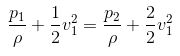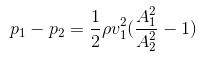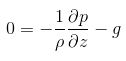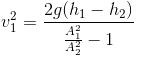# A venturimeter is used to

Venturimeter measures the flow speed of the incompressible fluid.

Venturi meter is named after an Italian physicist, Giovanni Battista Venturi.

There are two equations that are used for calculating the pressure difference between the downstream flow and the narrow pipe area and they are:

• Bernoulli’s theorem
• Continuity equation
• Euler equation

Bernoulli’s theorem:It can be seen that gravity is not taken into account as the flow is horizontal.

Continuity equation:

A1v1 = A2v2

Combining Bernoulli’s theorem and continuity equation we can find the pressure difference using the below equation:Euler equation is used for determining the pressure difference between the two locations:Using the above equation, the flow velocity can be determined:(0)(0)